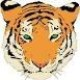# VB script to convert a number to a letter FollowAndy Halsema

I have a database field for an item's Cost that I want to print on the label. However, cost needs to be encoded so that a customer does not see what the item cost is. The numeric values would be encoded as such:

1 = W

2 = O

3 = R

4 = K

5 = U

6 = N

7 = I

8 = T

9 = E

10 = 11Try this

## Value = NumConv(Value)

You will need to make the field a Visual Basic Script > Event Controlled Script and as the data is coming from a database I would suggest the OnNewRecord option from the column on the left.

For the second line type the Value = and then in the column on the right double click on your database field with the number in. I used a Named Data Source for my testing and so the code will be slightly different fro you.

I hope this helps